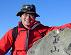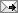2005/04/14

# 一位網友對新制退休金的指正

• 若每年初提撥C元，每年薪資成長G%，每年報酬率R%
1. FV = C(1+R)^(N)+C(1+G)*(1+R)^(N-1)+…+C(1+G)^(N-1)(1+R)
2. [(1+G)/(1+R)]FV = C(1+G)*(1+R)^(N-1)+C(1+G)^2*(1+R)^(N-2)+…+C(1+G)^N

FV=C*(1+R)*((1+R)^N-(1+G)^N)/(R-G)

• 若每年末提撥C元，每年薪資成長G%，每年報酬率R%
1. FV = C(1+R)^(N-1)+C(1+G)*(1+R)^(N-2)+…+C(1+G)^(N-1)
2. [(1+G)/(1+R)]FV = C(1+G)*(1+R)^(N-2)+C(1+G)^2*(1+R)^(N-3)+…+C(1+G)^N/(1+R)

FV=(C)*((1+R)^N-(1+G)^N)/(R-G)【廣為轉寄文章